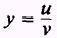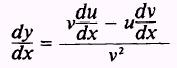Custom SearchQUOTIENTS Theorem 5. At a point where the denominator is not equal to zero, the derivative of the quotient of two differentiable functions of x is equal to the denominator times the derivative of the numerator minus the numerator times the derivative of the denominator, all divided by the square of the denominator. IfthenEXAMPLE. Find the derivative of the functionSOLUTION.- The derivative of the numerator is 2x, and the derivative of the denominator is 2. Therefore,PRACTICE PROBLEMS: Find the derivatives of the following:ANSWERS:Integrated Publishing, Inc. - A (SDVOSB) Service Disabled Veteran Owned Small Business